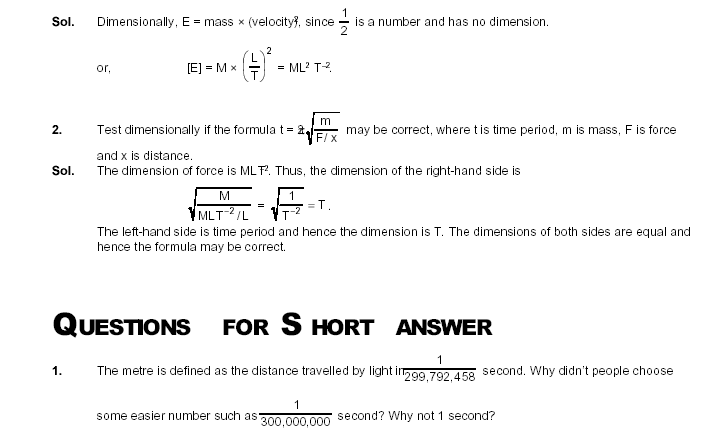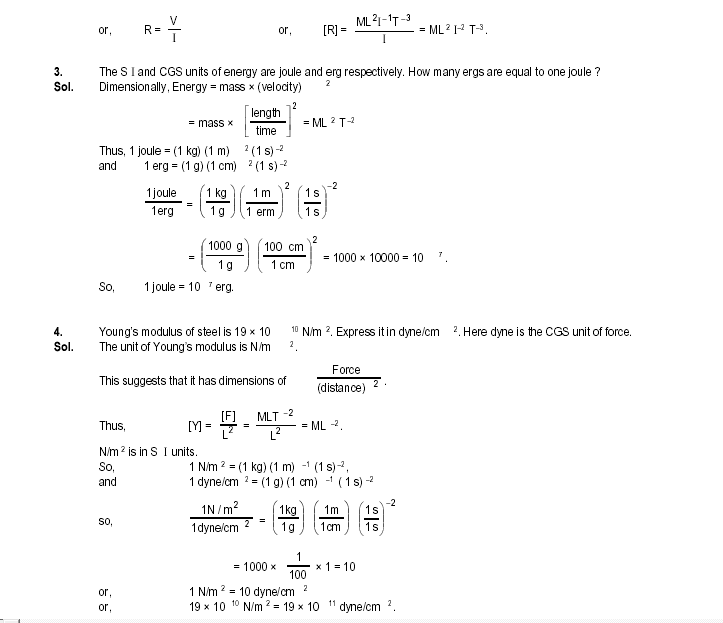## H C VERMA PHYSICS BOOK SOLUTIONS INTRODUCTION

 Solved Examples              1. Calculate the dimensional formula of energy from the equation E=1/2 mv2.2. What are the dimensions of :                 (a) volume of a cube of edge a,                (b) volume of a sphere of radius a,                (c) the ratio of the volume of a cube of edge a to the volume of a sphere of radius a?             3. Suppose you are told that the linear size of everything in the unverse has been doubled overnight. Can you test this statement by measuring sizes with a metre stick? Can you test it by using the fact that the speed of light is a universal constant and has not changed? What will happen if all the clocks in the universe also start running at half the speed? 4. If all the terms in an equation have same units, is it necessary that they have same dimensions? If all the terms in an equaiton have same dimensions, is it neccessary that they have same units? 5. If two quantities have same dimensions, do they represent same physical content? 6. It is desirable that the standards of units be easily available, invariable, indestructible and easily reproducible. If we use foot of a person as a standard unit of length, which of the above features are present and which are not? 7. Suggest a way to measure : (a) the thickness of a sheet of paper. (b) the distance between the sun and the moon.5. If velocity, time and force were chosen as basic quantities, find the dimensions of mass.

Sol. Dimensionally,              Force = mass × acceleration.